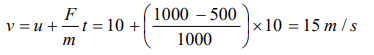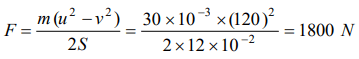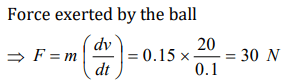## Laws of Motion Questions and Answers Part-7

1. An army vehicle of mass 1000 kg is moving with a velocity of 10 m/s and is acted upon by a forward force of 1000 N due to the engine and a retarding force of 500 N due to friction. What will be its velocity after 10 s
a) 5 m/s
b) 10 m/s
c) 15 m/s
d) 20 m/s

Explanation:2. A body of mass 2 kg is moving with a velocity 8 m/s on a smooth surface. If it is to be brought to rest in 4 seconds, then the force to be applied is
a) 8 N
b) 4 N
c) 2 N
d) 1 N

Explanation:3. The apparent weight of the body, when it is travelling upwards with an acceleration of $2 m\diagup s^{2}$ and mass is 10 kg, will be
a) 198 N
b) 164 N
c) 140 N
d) 118 N

Explanation: R = m(g + a) = 10 * (9.8 + 2) = 118 N

4. A man measures time period of a pendulum $\left(T\right)$ in stationary lift. If the lift moves upward with acceleration , $\frac{g}{4}$ then new time period will be
a) $\frac{2T}{\sqrt{5}}$
b) $\frac{\sqrt{5}T}{2}$
c) $\frac{\sqrt{5}}{2T}$
d) $\frac{2}{\sqrt{5}T}$

Explanation:5. A 30 gm bullet initially travelling at 120 m/s penetrates 12 cm into a wooden block. The average resistance exerted by the wooden block is
a) 2850N
b) 2200 N
c) 2000N
d) 1800 N

Explanation:6. A force of 10 Newton acts on a body of mass 20kg for 10 seconds. Change in its momentum is
a) 5 $kgm\diagup s$
b) 100 $kgm\diagup s$
c) 200 $kgm\diagup s$
d) 1000 $kgm\diagup s$

Explanation: dp = F*dt = 10 * 10 = 100 kg m/s

7. A body of mass 1.0kg is falling with an acceleration of $10 m\diagup sec^{2}$ . Its apparent weight will be $\left( g = 10 m\diagup sec^{2}\right)$
a) 1.0kgwt
b) 2.0kgwt
c) 0.5kgwt
d) Zero

Explanation: R = m(g−a) = m(10−10) = zero

8. A player caught a cricket ball of mass 150 gm moving at the rate of 20 m/sec. if the catching process be completed in 0.1 sec the force of the blow exerted by the ball on the hands of player is
a) 0.3 N
b) 30 N
c) 300 N
d) 3000 N

Explanation:9. If rope of lift breaks suddenly, the tension exerted by the surface of lift (a = acceleration of lift)
a) mg
b) $m\left(g+ a\right)$
c) $m\left(g- a\right)$
d) 0

10. A boy whose mass is 50kg stands on a spring balance inside a lift. The lift starts to ascent with an acceleration of $2 m s^{-2}$  . The reading of the machine or balance $\left(g=10 m sec^{-2}\right)$